Texas Go Math Grade 2 Lesson 12.3 Answer Key Write Multiplication Stories

Refer to our Texas Go Math Grade 2 Answer Key Pdf to score good marks in the exams. Test yourself by practicing the problems from Texas Go Math Grade 2 Lesson 12.3 Answer Key Write Multiplication Stories.

Texas Go Math Grade 2 Lesson 12.3 Answer Key Write Multiplication Stories

Explore

Use counters to model the problem. Draw what you modeled. Then write an addition sentence for the problem.Carlos has ___________ blocks.Sam has ___________ blocks

FOR THE TEACHER • Read the following two problems. For each problem, have children model with counters and then draw a picture and show the addition. Carlos has 2 blue blocks, 3 red blocks, and 5 green blocks. How many blocks does Carlos have? Sam has 5 blue blocks. 5 red blocks, and 5 green blocks. How many blocks does Sam have?
Carlos has 10 blocks.
Carlos has 2 blue blocks,
3 red blocks,
and 5 green blocks.
2 + 3 + 5 = 10
10  blocks that Carlos have
Sam has 15 blocks
Sam has 5 blue blocks
. 5 red blocks,
and 5 green blocks
5 + 5 + 5 = 15
15 blocks that Sam have

Math Talk
Mathematical Processes

Describe how the two problems are alike and how they different.
The above problems are same

Model and Draw

This story is about equal groups. The picture shows the story.Share and Show

Write stories about equal groups of toys. Use counters to show the groups. Draw a picture for each story.

Question 1.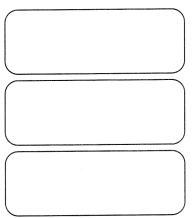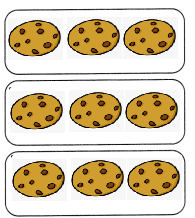Explanation:
3 x 3 = 9

Question 2.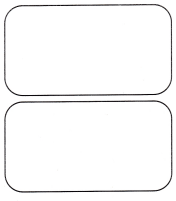Explanation:
Diana has 8 balloons shared among 2 people
4 x 2 = 8 balloons

Problem Solving

Write stories about equal groups of marbles. Use counters to show the groups. Draw a picture for each story.

Question 3.Explanation:
There are 12 marbles
shared among 6 people
each person got 2 marbles each
2 x 6 = 12

Question 4.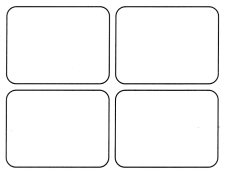Explanation:
There are 16 marbles shared among
4 members
4 x 4 = 16
Each person got 4 marbles.

Solve.

Question 5.
H.O.T. Multi-Step Drew has 2 bags with 10 marbles in each bag. Tony has 5 bags with 4 marbles in each bag. Use words to compare the number of marbles that Drew and Tony each have.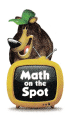20 marbles each have
Explanation:
Drew has 2 bags with 10 marbles in each bag.
2 x 10 = 20
Tony has 5 bags with 4 marbles in each bag.
4 x 5 = 20
same is the number of marbles that Drew and Tony each have.

Question 6.
Connect Mary Ann sees 4- starfish like the one shown. Look at the picture of a starfish. Which sentence best describes what she sees?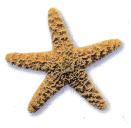(A) She sees 4 starfish with 5 legs each.
(B) She sees 5 starfish with 4 legs each.
(C) She sees 4 starfish with 3 legs each.
Explanation:
Ann sees 4- starfish like the one shown.
She sees 4 starfish with 5 legs each.
is the sentence best describes what she sees

Question 7.
Write a story about equal groups of apples. Use counters to show the groups. Draw a picture for your story.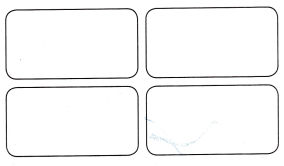Explanation:
There are 12 apples shared among 4 people
each person got 3 apples each
4 x 3 = 12

Question 8.
TEXAS Test Prep Which sentence best matches the picture?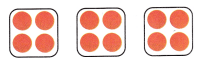(A) There are 3 groups of 4 counters each.
(B) There are 3 groups of 3 counters each.
(C) There are 7 groups of 2 counters each.
Explanation:
There are 3 groups of 4 counters each.
is the sentence best matches the picture

TAKE HOME ACTIVITY • Ask your child to show how he or she solved on exercise in the lesson.
Explanation:

Texas Go Math Grade 2 Lesson 12.3 Homework and Practice Answer Key

Write stories about equal groups of shells. Draw a picture for each story.

Question 1.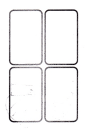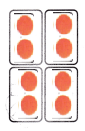Explanation:
There are 8 balls shared among 4 persons
4 x 2 = 8

Question 2.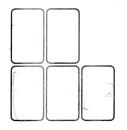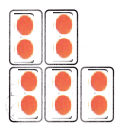Explanation:
There are 10 balls in the ground
they are shared among 5 players
2 x 5 = 10

Problem Solving

Question 3.
Complete the story. There are 7 circles in a row. There are 3 rows.
So, _____________
21 circles
Explanation:
There are 7 circles in a row.
There are 3 rows.
7 x 3 = 21
21 circles in 3 rows

Solve. Write or draw to explain.

Question 4.
Multi-Step Jose has 2 bags with 6 marbles in each bag. Ray has 3 bags with 4 marbles in each bag. Compare the number of marbles that Jose and Ray have using words.
12 marbles each have
Explanation:
Jose has 2 bags with 6 marbles in each bag.
2 x 6 = 12
Ray has 3 bags with 4 marbles in each bag.
3 x 4 = 12
12 = 12
same is the number of marbles that Jose and Ray have using words.

Lesson Check

Question 5.
Which sentence best describes the picture?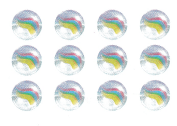(A) 3 children have 3 marbles each.
(B) 4 children have 3 marbles each.
(C) 4 children have 4 marbles each.
Explanation:
4 children have 3 marbles each.
is the sentence best describes the picture

Question 6.
There are 3 different cages of bunnies in the barn. Each cage has 8 bunnies in it. How many bunnies are in the barn?
(A) 21
(B) 30
(C) 24
Explanation:
There are 3 different cages of bunnies in the barn.
Each cage has 8 bunnies in it.
8 x 3 = 24
24 bunnies are in the barn

Question 7.
There are 10 chickens. Each chicken has 2 feet. How many feet are there?
(A) 20
(B) 12
(C) 22
Explanation:
There are 10 chickens.
Each chicken has 2 feet.
10 x 2 = 20
20 feet are there

Question 8.
Nicole saw some crayon boxes on a table. Which sentence best describes what she sees?(A) 10 boxes have 2 crayons in them.
(B) 4 boxes have 10 crayons in them.
(C) 2 boxes have 10 crayons in them.